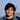# Negative Zero in JavaScript

Classical mathematics has no notion of a negative zero. Zero is often termed neither positive nor negative. In computing, however, there is a concept of signed zero.

JavaScript uses the IEEE 754 standard to represent numbers, which results in the language having a `-0` and `0`.

The IEEE 754 standard for floating point arithmetic (presently used by most computers and programming languages that support floating point numbers) requires both +0 and −0. The zeroes can be considered as a variant of the extended real number line such that 1/−0 = −∞ and 1/+0 = +∞, division by zero is only undefined for ±0/±0 and ±∞/±∞.

Although we often don’t distinguish between `-0` and `0` in everyday programming, it might be useful for rare cases. For example, a negative zero can be used to express a sense of direction (left or right, up or down). This could also be helpful in representing a moving object on a map (x and y coordinates).

There are certain quirks when working with negative zeros in JavaScript which I’ll try to summarize in this post.

## Equality

Although `-0` and `0` should be treated different, the conventional equality operators `==` and `===` treat them as same (according to the rules of equality).

``````const a = 0;
const b = -0;

console.log(a == b); // true
console.log(a === b); // true``````

In order to correctly compare `-0` and `0` we should use the `Object.is()` method. `Object.is()` unlike the `==` operator, doesn’t coerce and unlike the `===` operator doesn’t treat values differently.

``````const a = 0;
const b = -0;

console.log(Object.is(a, b)); // false``````

`Object.is()` unlike `==` and `===` also treats `NaN` as same.

``````console.log(NaN == NaN); // false
console.log(NaN === NaN); // false

console.log(Object.is(NaN, NaN)); // true``````

## Sign

`Math.sign()` can be used to determine the sign of a number. It also exhibits a different behavior when working with zeroes.

``````// for non zero values
console.log(Math.sign(13)); // 1
console.log(Math.sign(-13)); // -1

// for zeroes
console.log(Math.sign(0)); // 0
console.log(Math.sign(-0)); // -0``````Personal blog of Divyanshu Maithani. I’m a JavaScript engineer working with React, React Native, GraphQL and Node. I also create programming videos with my friend. In my spare time I play music and DoTA.